# Problems and solutions in calculating quality-adjusted life years (QALYs)

## Abstract

The quality-adjusted life-year (QALY) is a measure of the value of health outcomes. Since health is a function of length of life and quality of life, the QALY was developed as an attempt to combine the value of these attributes into a single index number. The QALY calculation is simple: the change in utility value induced by the treatment is multiplied by the duration of the treatment effect to provide the number of QALYs gained. QALYs can then be incorporated with medical costs to arrive at a final common denominator of cost/QALY. This parameter can be used to compare the cost-effectiveness of any treatment.

Nevertheless, QALYs have been criticised on technical and ethical grounds. A salient problem relies on the numerical nature of its constituent parts. The appropriateness of the QALY arithmetical operation is compromised by the essence of the utility scale: while life-years are expressed in a ratio scale with a true zero, the utility is an interval scale where 0 is an arbitrary value for death. In order to be able to obtain coherent results, both scales would have to be expressed in the same units of measurement. The different nature of these two factors jeopardises the meaning and interpretation of QALYs. A simple general linear transformation of the utility scale suffices to demonstrate that the results of the multiplication are not invariant.

Mathematically, the solution to these limitations happens through an alternative calculation of QALYs by means of operations with complex numbers rooted in the well known Pythagorean theorem. Through a series of examples, the new calculation arithmetic is introduced and discussed.

## Introduction

### The evolution of the concept of health

Traditionally, the health of populations has been measured using epidemiological indicators, including the presence/absence of disease and/or death (e.g. morbidity and mortality) . These classical indicators represent the paradigm of a theoretical model, devised ex professo, which help us to understand the complex reality implied by the term "health". This model, which is generally referred to as the "biomedical model", focuses on aetiological agents, pathological processes and biological, physiological and/or clinical results. The main aim of this model is to understand the mechanisms causing disease so as to be able to guide physicians in diagnosing and treating the disease .

Although these epidemiological indicators are extremely useful in depicting population health, by estimating life expectancy and identifying the causes of death, relatively recent changes in the way health is conceptualised have also led to changes in the way health is measured and the type and quantity of information gathered. This transformation is to a large extent the result of scientific and technical advances in medicine and improved living conditions in terms of housing, hygiene and food. These changes have led to increases in life expectancy and changes in the dominant pattern of morbidity, with the focus shifting from highly-lethal acute diseases to disabling chronic conditions. When coupled with the World Health Organization's (WHO) 1947 definition of health as "...not merely the absence of disease [but] also physical, mental and social welfare" , these changes denoted the beginning of a period in which health assessment has gone beyond the gathering of data on the presence/absence of disease and the quantification of individuals' "amount of life". New "psycho-social" models have been introduced in which consideration is also given to the need to individuals' "Quality of Life" .

While the intellectual and methodological foundations of the bio-medical model are rooted in disciplines such as biology, biochemistry and physiology, the new psycho-social model is founded in sociology, psychology and economics. By moving away from a purely biological model, the overall concept of health is enriched and a need arises to focus on areas such as the individual's ability to operate in society, disability, access to health services or the individuals' subjective perception of general well-being, among others.

### The integrating role of QALYs

In an attempt to integrate the biomedical and psycho-social models, a new approach has been proposed which can be labelled the bio-psycho-social model [5, 6]. The aim of this model is to combine the biological, individual and societal perspectives of health in a coherent fashion. A paradigmatic indicator within this model is the quality-adjusted life-year (QALY), which serves as a composite indicator allowing quality and quantity of life to be combined in a single index .

The possibility of combining quantity and quality of life in a single index can be combined is based on the idea that the quality of life can be quantified by applying the concept of "utility" , a concept rooted in the school of political philosophy known as utilitarianism. Consumer Choice Theory likewise describes how consumers decide what to buy on the basis of two fundamental elements: their budget constraints and their preferences. Consumer preferences for different consumables are also often represented by the concept of "utility" .

Within health and health care, the greater the preference for a particular health state, the greater the "utility" associated with it. "Utilities" of health states are generally expressed on a numerical scale ranging from 0 to 1, in which 0 represents the "utility" of the state "Dead" and 1 the utility of a state lived in "perfect health". The utilities assigned to a specific state of health can be estimated using a series of techniques such as Standard Gamble, Time Trade-Off or Rating Scale, or by means of pre-scored health state sorting systems (i.e. HUI, EQ-5D) .

The basic idea underlying the QALY is simple: it assumes that a year of life lived in perfect health is worth 1 QALY (1 Year of Life × 1 Utility = 1 QALY) and that a year of life lived in a state of less than this perfect health is worth less than 1. In order to determine the exact QALY value, it is sufficient to multiply the utility value associated with a given state of health by the years lived in that state. QALYs are therefore expressed in terms of "years lived in perfect health": half a year lived in perfect health is equivalent to 0.5 QALYs (0.5 years × 1 Utility), the same as 1 year of life lived in a situation with utility 0.5 (e.g. bedridden) (1 year × 0.5 Utility) .

### The application of QALYs in the economic analysis of health-care activities

Over the last two decades, QALYs have become increasingly widely used as a measure of health outcomes. This is largely due to three important characteristics. Firstly, the QALY combines changes in morbidity (quality) and mortality (amount) in a single indicator. Secondly, QALYs are easy to calculate via simple multiplication, although the prior estimation of utilities associated with particular health states is a more complicated task. Finally, QALYs form an integral part of one particular type of economic analysis within health-care, i.e. cost-utility analysis (CUA) .

Whereas in Cost-Effectiveness Analysis (CEA), incremental effects are assessed in natural units such as lives saved, years of life gained, blood pressure measured in mm of Hg, etc., in CUA the incremental improvements in health are measured using QALYs. A further advantage of QALYs, is that they allow the effectiveness and cost-effectiveness (or cost-utility) of interventions applied in very different disease areas to be compared, even when, because of their different outcomes, they would not be comparable within a CEA .

Table 1 shows the costs and outcomes, expressed in QALYs, generated by two alternative treatments (A and B) for a given medical condition. In a cost-utility analysis, costs and outcomes are compared by dividing the incremental cost by the incremental outcome of one treatment over the other, which will indicate how much each additional QALY gained with the new treatment will cost. In the case of the figures in Table 1, the cost-utility ratio is 192.31 dollars per additional QALY gained with treatment A. Incremental QALYs are often pictured as the difference in the rectangular areas resulting from the multiplication of life-years and utility. The QALYs from table 1 are shown graphically in Figure 1.

### The fallacy of the multiplicative QALY model

Despite the advantages of using a single indicator to measure the effectiveness of health-care interventions, QALYs have been widely criticized on ethical, conceptual and operational grounds [10, 11]. It has frequently been suggested, for example, that there is no sound theoretical basis for using QALYs nor for assuming that the social value of health states is no more than the simple sum or unweighted average of individual preferences obtained using techniques such as the Standard Gamble, Time Trade-off, etc. . Despite this, and despite the fact that a range of alternatives such as Healthy-Year Equivalents (HYEs), Disability-Adjusted Life Years (DALYs) or Person Trade-Offs (PTOs) , have been proposed to replace QALYs, none have so far succeeded in displacing the intuitively attractive QALY.

One area of QALY calculations which has received little attention is the multiplicative model which underlies the generation of QALY values. A potential flaw in this model is that utility values are used in the equation as if they were obtained on a ratio, and not on an interval scale, whereas they are in fact interval values . An interval scale is one in which the rank-ordering of objects is known with respect to an attribute and it is known how far apart the objects are from one another with respect to the attribute, but no information is available about the absolute magnitude of the attribute for any object . In the case of a ratio scale, the absolute values of points on a scale, and not just the intervals between them, are also meaningful, and can, for example, be multiplied.

The way in which QALYs are calculated can also be understood geometrically. The points in Figure 2 indicate two co-ordinates in a Cartesian plane limited by two axes representing years of life along the horizontal axis and utility along the vertical axis. Each of the points or co-ordinates represents the number of years lived in a state of health with a specified utility: co-ordinate A(1,0.5) is one year of life lived with a utility of 0.5, co-ordinate B(2,1) corresponds to 2 years of life lived with utility 1. The determination of the numerical value of the QALY associated with each of these points is carried out, according to the multiplicative model, by multiplying the value of the sides meeting at the vertex formed by these points. In this way, co-ordinates A and B are equivalent to 0.5 and 2 QALYs respectively. Therefore, going from health state A to B implies multiplying the value of the QALY by 4 (B/A = 4).

While this geometrical (multiplicative) model, which is that normally used to calculate QALYs may be of use in some applications (e.g. in calculating areas), it is not appropriate for assigning numbers to points located in a bi-dimensional space1 (i.e. a space in which the coordinates of two different points are calculated on different types of scales) . The ratios between the numerical values assigned by the multiplicative model do not correspond to the ratios of the distances between the elements (points or co-ordinates) on the plane.

This can be better understood through a simple linear transformation of the numerical scale of the utilities. The vertical axis in Figure 3 shows a transformation Y' of the original utilities (Y) from Figure 2 by means of the operation Y' = 2Y+1. Here, the value 0 is transformed into 1, 0.5 into 2, and 1 into 3. The type of transformation proposed (Y' = cY+b) is the only one which maintains the properties of the original scale .

The co-ordinates A' and B' in Figure 3 correspond to the same co-ordinates shown in Figure 2; the only difference is that the utilities (Y') are expressed on a scale running from 1 to 3 instead of from 0 to 1. If the multiplicative model is now applied to obtain the QALYs, the values for the co-ordinates will be A' = 2 and B' = 6. Paradoxically, whereas in Figure 2 there was a fourfold increase in QALY value, the increase in Figure 3 is smaller (B'/A' = 3), even though the position of the points is maintained.

This inconsistency highlights the fact that the ratios established between the numerical values (QALYs) assigned by the multiplicative model to the co-ordinates in the Cartesian plane do not correspond to the relationships that these elements have with each other in reality. The reason for this inconsistency is very simple: multiplication and division with interval scales are permissible only with respect to the intervals and not with respect to the scale values . Multiplying years of life by utilities makes no sense because the utilities are expressed on an interval scale.

### QALYs revisited: The Complex Number Model

Mathematically, a solution to these limitations of the multiplicative model is found in the Complex Numbers Model which is rooted in the well-known Pythagorean Theorem (if a triangle has sides of length a, b, c, with sides a, b enclosing an angle of 90 degrees then: a2 + b2 = c2) . The Theorem is of fundamental importance in Euclidean Geometry, where it serves as a basis for the definition of distance between two points. A complex number z is an ordered pair of real numbers (a, b) that has a representation-point P in a plane with co-ordinates (a, b) (Figure 4). The number a is called the real part of z while b is called the imaginary part of z. Two complex numbers are equal if and only if their real parts are equal and their imaginary parts are equal. The magnitude or modulus of a complex number z is denoted |z| and defined as the distance from 0 to P, that is: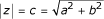The idea of a QALY is in fact similar to a complex number, as it is made up of a real part (Length of Life) and an imaginary part (Utility), in the sense that utilities are intangible and not susceptible to direct observation. The magnitude of the QALY cannot be calculated by multiplying both components but by determining the modulus of such complex composition. Thus, the QALY must be calculated as the square root of (length of life2 + utility2).

Unlike the multiplicative model, the Complex Number model for the calculation of the QALYs is based on operations implying the intervals separating the values on the utility and time scales (b and a, respectively) and not the values themselves. The QALYs thus obtained respect the spatial relationship between the numerical values assigned to the elements in the Cartesian plane. Figure 5 replicates the contents of Figure 2 but assigns the QALY values obtained from the Complex Number model to the A and B co-ordinates. The ratio of B/A in this case is equal to 2 (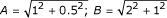). Figure 6 shows the transformation Y' of the original utilities (Y) by means of the operation Y' = 2Y + 1. We can see that A equals 1.414 (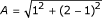) and B 2.828 (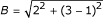), which provides the same value for the ratio B/A as that obtained before transforming the utility scale. The Complex Number model assigns numerical values to the elements in question so that these maintain the invariance of their ratios, thus respecting the attribute's bi-dimensionality.

Because the utility value lies between 0 and 1, the resulting distance obtained from the Complex Number approach may be dominated by the variable on the X axis (Length of Life) if it takes a large value (i.e. 75). One way around this problem is to similarly bound the length of life variable within 0–1 as well. Likewise, to set the maximum possible QALY value at 1, the c value initially obtained by this procedure must be divided by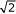= 1.4142 (as when a = 1 and b = 1 then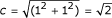). To reverse the standardization, the value resulting from this operation must be multiplied by the actual length of life. The new equation for calculating QALYs is therefore as follows: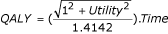Table 2 presents the QALYs corresponding to different values on the utility scale when the number of years remains constant and equal to 1. The most noteworthy aspect of this table is that, unlike in the multiplicative model, whenever utility reaches its minimum value the value of the resulting QALY is greater than 0. To some extent, it could be said that this new way of calculating the value of QALYs gives greater relative weight to the amount of life, making it impossible for this to be reduced to nullity. When dealing with the gains obtained by health care interventions, this seems appropriate as it adds additional weight to the mere fact of survival. For utilities expressed with a level of precision of 0.05 units, the table can be used to calculate any QALY; it is sufficient simply to multiply the QALY value in the last column by the number of years in question. For example, 2 years lived in a state of health with a utility of 0.5 would be worth 0.7906 × 2 = 1.58 QALYs, and 4 years lived with a utility of 0.7 would be worth 3.45 QALYs (0.8631 × 4).

Figure 7 illustrates with a solid line the function which, based on the Complex Number model, relates utility (Y-axis) and QALYs (X-axis) when time is set to 1 year. The function takes the form of a hyperbola in which the vertex is located at the co-ordinate (0.7071,0). Hyperbolic functions like this arise in many problems of mathematics and mathematical physics in which equations involving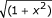appear . Figure 7 also illustrates with a broken line the function which relates utility and QALYs according to the multiplicative model; in this case, the function is linear and intersects the X-axis at the value 0, indicating that when the utility of a state of health is equivalent to 0, the value of the QALY will also be 0, regardless of the number of years lived. The notion that a patient gains no QALYs even when an intervention increases life expectancy/survival would be at the very least counterintuitive for many.

## Conclusions

QALYs are often represented as the rectangular area resulting from the product of their constituent factors: utility and time. But that is like comparing apples with oranges: quality (utility) and quantity (time) of life are of essentially different "data-types" and cannot be combined by a simple product of their numerical values. Contrary to what is assumed, the very nature of this arithmetical operation means that the resulting QALY value is not expressed in the same units as the time scale (years). The QALYs in the multiplicative model are in fact square units, i.e. units used to measure areas; they are not comparable to units of time. The Complex Number model, while capturing the dimensional nature of the time-utility composite, is expressed in linear units, which are also directly comparable to years of life on the time scale: the value of the complex number is equivalent to the distance between the co-ordinate (0,0) of the Cartesian plane and the representation-point P of this number (see Figure 4); the standardization of this distance by means of its division bymeans we are using the same units as the time scale. As Figure 2 showed, when the multiplicative model was applied, the ratio between co-ordinates B and A was equal to 4; however, when the Complex Number model was applied, the ratio between B and A was equal to 2 (Figure 5). In general, if the ratio of two lengths is b/a, the ratio of their areas is b2/a2. Thus the ratio is 2 for the Complex Number Model, and 4 (22) for the multiplicative model solution.

This article has pointed out, in addition, that the Complex Number model respects the bi-dimensional spatial relationships established between different combinations of time and utility when these are represented as points or co-ordinates on the Cartesian plane, thus making it a more suitable geometrical model than the multiplicative model for calculating QALYs. This new means of calculating QALYs does not mean they will be significantly more difficult to estimate, and they can be used and interpreted as before. Table 3, for example, shows the incremental QALYs pictured in table 1 with the new method of calculation based on the Complex Number model. In this case the new form of computing QALYs attenuates the QALY difference attributed to the intervention: from a previous increment of A over B of 2.6 QALYs, the increase is now 2.23 QALYs, implying, in the end, an increase in the cost-effectiveness ratio.

The new formulation of the QALYs is noteworthy for its less dramatic adjustment of years of life than that implied by the multiplicative model. The maximum penalization represented by living in a sub-optimal state of health is capped at 30% of the total time lived in that state. In the case of the multiplicative model, the penalization can reach 100%, a point that to some extent contradicts our deep-seated survival instinct. The disputed degree of validity and reliability of the measurement of utilities as well as the doubts over the possibility of extending it to all of the individuals in the population  are the greatest obstacles so far to assigning the same relative weight to the quality and quantity of life. By giving the QALY a real part (Length of Life) and an imaginary part (Utility), the Complex Number model provides an appropriate theoretical framework for combining time and health preferences into a single index, yet limiting the power of the latter to modulate the final value of the indicator.

QALYs continue to represent the paradigm of the integration of the bio-medical and the psycho-social models and this new approach to calculating QALYs may represent a further step in the integration of different health dimensions without giving up the use of mathematically sound procedures for this purpose.

## Note

In attempting to measure the physical attributes of objects or people, scientists and laypeople take care to measure just one attribute of the target at a time. This is because the measurement problem consists of developing procedures that would permit a number to be assigned to each quantity of the attribute in such a way that certain relations between the numbers reflect analogous relations between the quantities. This procedure is possible for those attributes whose quantities form a one-dimensional series. In this case, the attribute can be conceived as a one-dimensional continuum, analogous to the straight line in geometry. Assigning numbers to quantities is analogous to specification of the position of points on the straight line. This focus on one attribute or dimension is usually referred to as unidimensionality. For those attributes, like the QALY, whose quantities form a multidimensional series the measurement problem is more complex. Here the problem is to develop procedures to assign a set of numbers to each quantity (quality and quantity of life) so that the numbers, when considered in terms of a specified geometrical system, reflect relations among the various quantities. The number of numbers to be assigned to each quantity corresponds to the dimensionality of the attribute. Thus the analogy is now with a multidimensional geometrical space. Whereas in unidimensional measurement, the attribute corresponds to the straight line (a unidimensional space), and the quantity to a point on the line, in multidimensional measurement or scaling, the attribute corresponds to an n-dimensional space, and the quantity to a point in that space. Whereas the process of assigning numbers in unidimensional measurement or scaling corresponds to the location of points on a line, in terms of the order of the points, their distances from one another, and/or their distances from an origin, the process of assigning sets of numbers in multidimensional scaling corresponds to locating the points in a multidimensional space in terms of a set of relations between the points as specified by the particular geometrical model. The geometrical models that might be employed are, of course, of many different varieties. Besides the metric spaces, such as the Euclidean model, other non-Euclidean models might be used .

Regardless of the particular model used, the nature of the problem remains essentially the same. The meaning of the numbers assigned to the elements in the model is specified by the model. Rules of correspondence are established, relating elements and properties of the model to observable data, thus converting the model into a testable theory. If the theory is verified, numbers are assigned to the quantities of the multidimensional attribute as specified by the theory. Once this is done, the multidimensional attribute in question has been measured .

## References

1. Hennekens CH, Buring JE: Epidemiology in Medicine Little, Brown & Co.: Boston 1987.

2. World Health Organization: International Statistical Classification of Diseases and Related Health Problems (10th Revision) WHO: Geneva 1989.

3. World Health Organization: The constitution of the World Health Organization. WHO Chronicle 1947, 1: 6–24.

4. Testa MA, Simonson DC: Assessment of Quality-of-Life Outcomes. N Eng J Med 1996, 334: 835–840. 10.1056/NEJM199603283341306

5. Wilson IB, Cleary PD: Linking Clinical Variables With Health-Related Quality Of Life. A conceptual Model of Patient Outcomes. JAMA 1995, 273: 59–65. 10.1001/jama.273.1.59

6. World Health Organization: International Classification of Functioning, Disability and Health WHO: Geneva 2001.

7. Patrick DL, Erickson P: Health Status And Health Policy. Oxford University Press: New York, NY 1993.

8. Drummond MF, O'Brien B, Stoddart GL, Torrance GW: Methods for the Economic Evaluation of Health Care Programmes. 2 Edition Oxford Medical Publications: Oxford 1997.

9. Mankiw NG: Principles of Economics Harcourt Brace & Co.: Boston 1998.

10. Nord E: Cost-Value Analysis in Health Care: Making Sense out of QALYs Cambridge University Press: Cambridge 1999.

11. Duru G, Auray JP, Beresniak A, Lamure M, Paine A, Nicoloyannis N: Limitations of the methods used for calculating quality-adjusted life-year values. Pharmacoeconomics 2002, 20: 463–73.

12. Torgerson WS: Theory and Methods of Scaling John Wiley and Sons: New York 1958.

13. Nunnally JC, Bernstein IH: Psychometric Theory Mcgraw-Hill: New York 1994.

14. Macran S, Kind P: "Death" and the valuation of health-related quality of life. Med Care 2001, 39: 212–227.

15. Wells D: The Penguin Dictionary of Curious and Interesting Geometry Penguin: London 1991, 106–109.

16. Lockwood EH: The Hiperbola. In: A Book of Curves Cambridge University Press: Cambridge 1967, 24–33.

## Acknowledgements

Our thanks to Dr. Michael Herdman, for his careful reading of the manuscript and helpful suggestions; an to Dr. Alfonso Casado, for his contribution to an early phase of this work.

## Author information

Authors

### Corresponding author

Correspondence to Luis Prieto.

### Authors' contributions

LP and JAS actively participated in the discussions that lead to the present manuscript. Both authors participated in writing the manuscript and read and approved the final one.

## Authors’ original submitted files for images

Below are the links to the authors’ original submitted files for images.

## Rights and permissions

Reprints and Permissions

Prieto, L., Sacristán, J.A. Problems and solutions in calculating quality-adjusted life years (QALYs). Health Qual Life Outcomes 1, 80 (2003). https://doi.org/10.1186/1477-7525-1-80